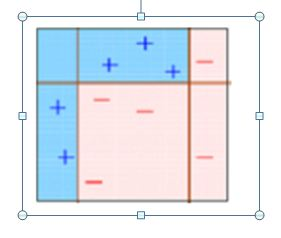/  Technology   /  Ensemble Learning Techniques in Machine Learning## Ensemble Learning Techniques in Machine Learning

Let’s understand the ensemble learning, suppose you are a director of a movie and you created a short movie on a very interesting topic and now you want to know the feedback of the movie before release. Here we are having two ways to know the review.

2. You can ask 5 colleagues of yours. This will provide a better idea about your movie and some people are not aware of your subject of your movie.

3. Asking 50 people to rate the movie. In this some of your friends and some may be your colleagues. In this case you would be more generalized because you are having different sets of skills.

From the above examples, you can make the better decisions as compared to individuals. This can be done by Machine Learning algorithm called Ensemble Learning.

In this we are have various techniques they are:

1. Simple ensemble techniques

## Simple ensemble techniques:

### Max Voting:

This method is mainly used for the classification. In this we are using multiple models to make the predictions for each datapoint. In this we consider each model is as vote.  The predictions which we are getting from the models are used as final prediction.

For example:

You are asked 5 colleagues to give the review about your movie we will assume three of five rated as 3 and two of them gave 4. Since the majority of the given rating is 4. Then the final rating will be taken as 4.

The result of max voting is:## Averaging:

The Averaging is similar to the maxvoting , but in this multiple prediction are done by each data point in averaging. In this method we can take average of all the models and make the final prediction. It is used for both regression and classification problems.

For example:

In the averaging method would take the average of all the values.

i.e. (3+4+3+4+3)/5 = 3.4## Weighted Average:

This is the extended version of averaging method in this every model is assigned different weights which defines the importance of each model for the predictions.

For example:

From your friends three friends don’t have the much knowledge about this field and others are critics then these two friends are given most important compared to others.

Then the result is,

[(5*0.23) + (4*0.23) + (5*0.18) + (4*0.18) + (4*0.18)] = 4.41.### Stacking:

This is one type of ensemble learning technique which make the predictions from the multiple models.

For example:

knn , svm and decision tree to build a new model. The predictions are mainly depends on test data set. In this we can easily create multiple models.

### Blending:

This blending technique is very similar to the stacking but it uses only validation set from the training set. In other words the predictions are mainly done on the validation set and the validation set and predictions are used to build a model and that model will run on test set.

### Bagging:

The bagging technique tells us to combine all the models to get the generalized result. But here we are using the same data for all the models then there is a high chance to get the same result from all the models then what’s the use of this?  That’s why we can use bootstrap sampling technique to create the multiple subsets from the original data with replacement.

Bagging or bootstrap aggregation is an ensemble technique that uses the sample datasets. These sample datasets are non other than the part of or the subset of original dataset.In this bagging

1. We can create a multiple subsets and selecting the observations with replacement.

2. Create a base model on each subset.

3. These all models are independent with each other and run in parallel.

4. Combine all these models to get the final predictions.## Boosting:

Boosting is a sequential process, each subsequent model correct the predictions of previous model and the subsequent models are dependent on the previous models.

Basically, the boosting algorithm is done like this

1. We create a subset from the original dataset.

2. All data points are given equal weights.

3. Create the base model for the subset

4. The model is used to make the predictions on the total data set.5. Now errors are calculated by using the actual and predicted values.

6. If you find any in corrected prediction, that gets higher weights. The 3 misclassified blue points will give higher weights.

7. For that misclassified we can create another model to reduce that error.8. And now create multiple models to reduce the error in the previous model.

9. And the final model is combining all the weak models to get the weighted mean of all models.10. Individual learners are not performing well on the entire dataset they perform well only on some part of the dataset that’s why we can use the Boosting algorithm to combine all weak learners and form a strong learner.